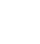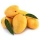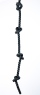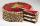# Fraction calculator

The calculator performs basic and advanced operations with fractions, expressions with fractions combined with integers, decimals, and mixed numbers. It also shows detailed step-by-step information about the fraction calculation procedure. Solve problems with two, three, or more fractions and numbers in one expression.

## Result:

### 11/4 - 1/3 = 11/12 ≅ 0.9166667

Spelled result in words is eleven twelfths.

### How do you solve fractions step by step?

1. Conversion a mixed number 1 1/4 to a improper fraction: 1 1/4 = 1 1/4 = 1 · 4 + 1/4 = 4 + 1/4 = 5/4

To find a new numerator:
a) Multiply the whole number 1 by the denominator 4. Whole number 1 equally 1 * 4/4 = 4/4
b) Add the answer from previous step 4 to the numerator 1. New numerator is 4 + 1 = 5
c) Write a previous answer (new numerator 5) over the denominator 4.

One and one quarter is five quarters
2. Subtract: 5/4 - 1/3 = 5 · 3/4 · 3 - 1 · 4/3 · 4 = 15/12 - 4/12 = 15 - 4/12 = 11/12
For adding, subtracting, and comparing fractions, it is suitable to adjust both fractions to a common (equal, identical) denominator. The common denominator you can calculate as the least common multiple of both denominators - LCM(4, 3) = 12. In practice, it is enough to find the common denominator (not necessarily the lowest) by multiplying the denominators: 4 × 3 = 12. In the following intermediate step, it cannot further simplify the fraction result by canceling.
In other words - five quarters minus one third = eleven twelfths.

#### Rules for expressions with fractions:

Fractions - simply use a forward slash between the numerator and denominator, i.e., for five-hundredths, enter 5/100. If you are using mixed numbers, be sure to leave a single space between the whole and fraction part.
The slash separates the numerator (number above a fraction line) and denominator (number below).

Mixed numerals (mixed fractions or mixed numbers) write as integer separated by one space and fraction i.e., 1 2/3 (having the same sign). An example of a negative mixed fraction: -5 1/2.
Because slash is both signs for fraction line and division, we recommended use colon (:) as the operator of division fractions i.e., 1/2 : 3.

Decimals (decimal numbers) enter with a decimal point . and they are automatically converted to fractions - i.e. 1.45.

The colon : and slash / is the symbol of division. Can be used to divide mixed numbers 1 2/3 : 4 3/8 or can be used for write complex fractions i.e. 1/2 : 1/3.
An asterisk * or × is the symbol for multiplication.
Plus + is addition, minus sign - is subtraction and ()[] is mathematical parentheses.
The exponentiation/power symbol is ^ - for example: (7/8-4/5)^2 = (7/8-4/5)2

#### Examples:

subtracting fractions: 2/3 - 1/2
multiplying fractions: 7/8 * 3/9
dividing Fractions: 1/2 : 3/4
exponentiation of fraction: 3/5^3
fractional exponents: 16 ^ 1/2
adding fractions and mixed numbers: 8/5 + 6 2/7
dividing integer and fraction: 5 ÷ 1/2
complex fractions: 5/8 : 2 2/3
decimal to fraction: 0.625
Fraction to Decimal: 1/4
Fraction to Percent: 1/8 %
comparing fractions: 1/4 2/3
multiplying a fraction by a whole number: 6 * 3/4
square root of a fraction: sqrt(1/16)
reducing or simplifying the fraction (simplification) - dividing the numerator and denominator of a fraction by the same non-zero number - equivalent fraction: 4/22
expression with brackets: 1/3 * (1/2 - 3 3/8)
compound fraction: 3/4 of 5/7
fractions multiple: 2/3 of 3/5
divide to find the quotient: 3/5 ÷ 2/3

The calculator follows well-known rules for order of operations. The most common mnemonics for remembering this order of operations are:
PEMDAS - Parentheses, Exponents, Multiplication, Division, Addition, Subtraction.
BEDMAS - Brackets, Exponents, Division, Multiplication, Addition, Subtraction
BODMAS - Brackets, Of or Order, Division, Multiplication, Addition, Subtraction.
GEMDAS - Grouping Symbols - brackets (){}, Exponents, Multiplication, Division, Addition, Subtraction.
Be careful, always do multiplication and division before addition and subtraction. Some operators (+ and -) and (* and /) has the same priority and then must evaluate from left to right.

## Fractions in word problems:

• Difference between fractionsWhat is the difference when you take away 1/6 from 2/8?Sadie practiced her spelling words for 3/4 of an hour, and Max practiced his spelling words for 5/12 of an hour. In the simplest form, how much longer did Sadie practice than Max?
• Find the 24Find the difference between 2/7 and 1/21
• Cups of flourJade was baking cupcakes for her class. She has 4 5/4 cups of flour.  For one batch, she used 1 2/3 cups of flour.  On another batch, she used 7/9 of a cup of flour.  How much flour does Jade have left after making the two batches of cupcakes?
• Bitoo and ReenaBitoo ate 3/5 part of an apple and the remaining part was eaten by his sister Reena. How much part of an apple did Renna eat? Who had the larger share? By how much?
• Rita hasRita has 3/4 m of Ifugao cloth. She used 2/3 m for placement. What part of Ifugao cloth was left?A basket contains three types of fruits weighing 87/4 kg in all. If 23/4 kilograms of these are oranges, 48/7 kg are mangoes, and the rest are apples. What is the weight of the apples in the basket?From a rope that is 11 m long, two pieces of lengths 13/5 m and 33/10 m are cut off. What is the length of the remaining rope?Rodney has a board that is 5/6 yards long. He cuts 1/5 yard off the board and uses the rest of the board to make a frame. How much of the board is used to make the frame?Ari and Joey share a 30-ounce box of cereal. By the end of the week, Ari has eaten 3/10 of the box, and Joey has eaten 3/5 of the box of cereal. How many ounces are left in the box?A 14.5-gallon gasoline tank is 3/4 full. How many gallons will it take to fill the tank? Write your answer as a mixed number.To three-eighths of one third, we add five quarters of one half and multiply the sum by four. How much will we get?Thomas ate 1/3 of cake, Bohouš of the rest of the cake ate 2/5. What fraction of cake left over for others?